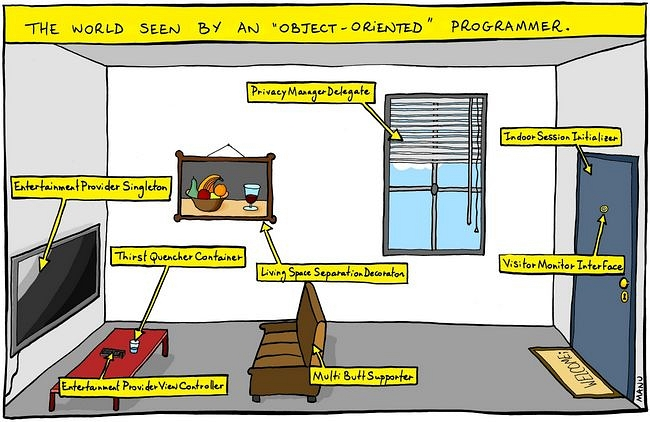Why are programs written in Java so verbose? The answer seems to be that Java does not support function pointers or functions as first class values.

For example, solving an ODE in Python proceeds as follows. You define a function for the derivative, and then pass that to the solver along with some initial conditions and other parameters. Easy enough.

The equivalent in Java (example taken from the Apache commons maths library) requires an entire class to be written, implementing the oddly named interface FirstOrderDifferentialEquations.

private static class CircleODE implements FirstOrderDifferentialEquations {

private double[] c;
private double omega;

public CircleODE(double[] c, double omega) {
this.c     = c;
this.omega = omega;
}

public int getDimension() {
return 2;
}

public void computeDerivatives(double t, double[] y, double[] yDot) {
yDot = omega * (c - y);
yDot = omega * (y - c);
}

}

// then in some other class...

FirstOrderIntegrator dp853 = new DormandPrince853Integrator(1.0e-8, 100.0, 1.0e-10, 1.0e-10);
FirstOrderDifferentialEquations ode = new CircleODE(new double[] { 1.0, 1.0 }, 0.1);
double[] y = new double[] { 0.0, 1.0 }; // initial state
dp853.integrate(ode, 0.0, y, 16.0, y); // now y contains final state at time t=16.0


This is a lot of work and obfuscates the problem at hand, the definition of a derivative and a call to a solver. Java seems to be unique in its pedantic style, at least among common languages in use today. In C one can use function pointers, Fortran can pass functions, Python has first-class functions, Haskell has higher-order functions, and so on.

It turns out that Java programmers are forced to do defunctionalization since Java does not support higher order functions. Here’s a quote from a blog post on plover.net:

Defunctionalization is a program transformation that removes the higher-order functions from a program. The idea is that you replace something like λx.x+y with a data structure that encapsulates a value of y somewhere, say (HOLD y). And instead of using the language’s built-in function application to apply this object directly to an argument x, you write a synthetic applicator that takes (HOLD y) and x and returns x + y. And anyone who wanted to apply λx.x+y to some argument x in some context in which y was bound should first construct (HOLD y), then use the synthetic applicator on (HOLD y) and x. </blockquote>

In Haskell we might implement yDot as:

The parameters c and omega are the slowest varying, so we put them before y. Since all functions in Haskell are curried, we can conveniently produce the function that we need by partially applying the c and omega values:

*Defun> :t yDot [1.0, 1.0] 0.1
yDot [1.0, 1.0] 0.1 :: [Double] -> [Double]


In this way yDot is a higher order function. To make it first-order we have to defunctionalize it. Following the example on plover.net we define a data structure to hole the c and omega values:

And we need a function to “apply” this value to get the actual yDot value.

Basically Hold and fakeApply are equivalent to the CircleODE class above.

Example:

Defunctionalization appears to be the cause of the excessive use of nouns in Java code, resulting in things like the Abstract Singleton Proxy Factory Bean, or the Abstract Factory design pattern.